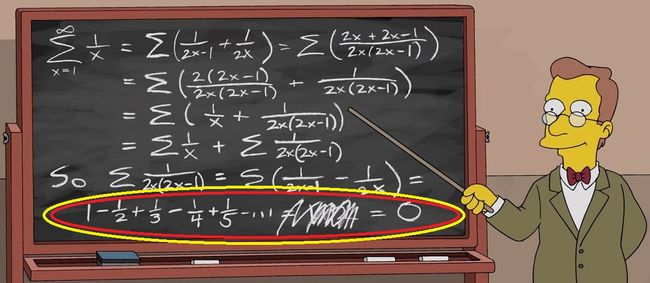# Log 2 equals to 0

Calculus Level 2Consider the harmonic series: $\displaystyle \sum_{x=1}^\infty \frac1x = \frac11 + \frac12 + \frac13 + \frac14 + \frac15 + \ldots$

I'm going to rearrange the terms to obtain $\ln(2) = 0$ by first grouping the terms in pairs:

\begin{aligned} \displaystyle \sum_{x=1}^\infty \frac1x &=& \left( \frac11 + \frac12 \right)+\left( \frac13 + \frac14 \right)+\left( \frac15 + \frac16 \right)+\ldots \\ \displaystyle &=& \sum_{x=1}^\infty \left( \frac1{2x-1} + \frac1{2x} \right) \\ \displaystyle &=& \sum_{x=1}^\infty \left( \frac{2x+2x-1}{2x(2x-1)} \right) \\ \displaystyle &=& \sum_{x=1}^\infty \left( \frac{2(2x-1) + 1}{2x(2x-1)} \right) \\ \displaystyle &=& \sum_{x=1}^\infty \left [ \frac{2(2x-1)}{2x(2x-1)} + \frac1{2x(2x-1)} \right] \\ \displaystyle &=& \sum_{x=1}^\infty \left [ \frac1x + \frac1{2x(2x-1)} \right] \\ \displaystyle \xcancel{\sum_{x=1}^\infty \frac1x} &=& \xcancel{\sum_{x=1}^\infty \frac1x} + \sum_{x=1}^\infty \frac1{2x(2x-1)} \\ \displaystyle 0 &=& \sum_{x=1}^\infty \frac1{2x(2x-1)} \\ \displaystyle 0 &=& \sum_{x=1}^\infty \left ( \frac1{2x} - \frac1{2x-1} \right ) \\ \displaystyle 0 &=& 1 - \frac12 + \frac13 - \frac14 + \frac15 - \ldots = \ln(2) \\ \end{aligned}

Above shows 10 steps for the supposed claim of $\ln(2) = 0$. How many of these steps are incorrect?